## Mathematics: Toolkit

### The Rule of Three

On the first page of his manuscript, Michael carefully states a commercial problem: "If 400 pounds of pepper is worth 49ducats, what is the value of 315 pounds?"

We recognize this as a problem of proportions, in which a is to b as c is to d. We might write this out as: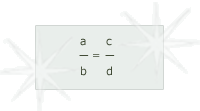Michael recognized his problem as classic material for what he called the "high fashion of the rule of three." According to this rule, if four numbers are related like those in his problem, three of the numbers could be used to find the fourth.

In modern math, we could solve the problem four ways to find any of the four numbers. But the rule of three used only one solution: You always multiplied the second and third terms in your problem, then divided by the first.

In modern notation, Michael's rule would be: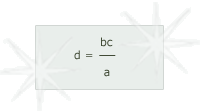Because he used only this one rule, but not modern notation, Michael had to be sure to state the problem in the right order. Then he could proceed to finding the value of his pepper in ducats, grossi (which he calls denari), and piccoli.

[Note: Click on the images to see the calculations in context.]
• a) The value in ducats

Michael begins by finding the value of his cargo in ducats.

• 1] He starts by writing out his numbers in the same order that he stated the problem: 400 49315.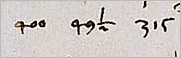• 2] Next he writes out the calculation he must perform after transforming 49intoto get rid of the inconvenient fraction. Note that the lines connecting the numbers are not related to modern mathematical notation. They show which numbers Michael intended to multiply together (400x2x1 and 1x92x315).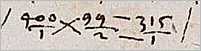• 3] Michael multiplies 400 by 2 by 1 to get 800 and keeps this answer in his head.

• 4] Multiplying 315 by 99 is more difficult. Michael has to write out this computation. On the left are the numbers to be multiplied. In the middle are the intermediate products. On the right is the answer of 31185.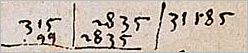• 5] To complete the rule of three, all Michael has to do is divide 31185 by 800. The division can be seen on the right of the page, but it almost looks as if Michael has divided 31185 by 8000. What we see here is the end result of Michael's galera (or "galley") method of division. To follow Michael's method of division, click on the calculation below: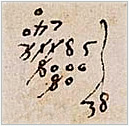Follow Michael's method of division »
• 6] Neither the decimal point nor decimal fractions existed in Michael's time, so he had to express the result of his division as a compound fraction, concluding that his cargo of 315 pounds of pepper is worth 38 and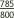ducats. The 785 is visible in the division above.

• b) The remainder in denari (grossi)

In addition to gold ducats, Venetians used silver coins called grossi (which Michael refers to as denari) and coins mainly of copper called piccoli. Each ducat was equal to 24 grossi, and each grosso was equal to 32 piccoli. This was another problem for the rule of three.

Michael wanted to know how muchducats would be worth in denari. He doesn't state the problem in words, but in modern notation it would be: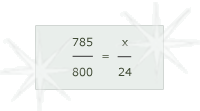and the solution would be: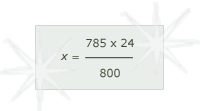Michael carries out exactly this computation.

• c) The remainder in piccoli

Finally, Michael wanted to know how much his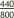denari, which Venetians normally referred to as grossi, was worth in piccoli, given 32 piccoli to the grosso.

This was his third use of the rule of three.

In modern notation the calculation would be it would be: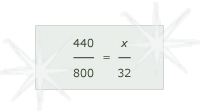and the solution would be: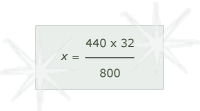Michael carries out exactly this computation.

And so in the end Michael is able to conclude that his 315 pounds of pepper is worth 38 ducats, 23 denari, and 17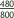piccoli.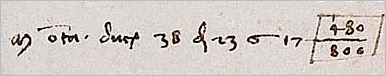See how Michael solves the problem using algebra or the rule of double false position.